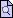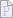# Ma 1025 mod 1 question 6-20

## Question 6

1 point

In chemistry the volume for a certain gas is given by V=25TV=25T, where VV is measured in cc and TT is temperature in °C°C. If the temperature varies between 90°C90°C and 110°C110°C , find the set of volume values.

Enter the exact answer in interval notation.

To enter ∞∞, type infinity. To enter ∪∪, type U.

The range of the volume iscc.

## Question 7

1 point

Describe all the xx-values within or including a distance of 44 units from the number 88.

To enter ∞∞, type infinity. To enter ∪∪, type U.

## Question 8

1 point

Write the set in interval notation.

{x|−4<x≤8}x|−4<x≤8

To enter ∞∞, type infinity. To enter ∪∪, type U.

## Question 9

1 point

Solve the inequality involving absolute value.

|x+4|≥−9x+4≥−9

Enter the exact answer in interval notation.

To enter ∞∞, type infinity. To enter ∪∪, type U.

## Question 10

1 point

Solve the inequality.

4x−10≤64x−10≤6

Enter the exact answer in interval notation.

To enter ∞∞, type infinity. To enter ∪∪, type U.

## Question 11

1 point

Solve the inequality.

−4(x−1)+2>2x−3−3x−4x−1+2>2x−3−3x

Enter the exact answer in interval notation.

To enter ∞∞, type infinity. To enter ∪∪, type U.

## Question 12

1 point

Solve the inequality involving absolute value.

|x−3|+4≥10x−3+4≥10

Enter the exact answer in interval notation.

To enter ∞∞, type infinity. To enter ∪∪, type U.

## Question 13

1 point

Solve the compound inequality.

2x−8<−142x−8<−14   or   7x+2≥97x+2≥9

Enter the exact answer in interval notation.

## Question 14

1 point

Solve the equation for xx.

5x+5=3x−85x+5=3x−8

Enter the exact answer. Improper fractions are acceptable solutions.

x=x=

## Question 15

1 point

Solve the equation for xx.

x+27−x−16=2x+27−x−16=2

x=x=

## Question 16

1 point

Solve the equation for x.

4(x+3)−42=184x+3−42=18

x=

## Question 17

1 point

Solve for xx.

6(x+3)−27=x+66x+3−27=x+6

x=x=

## Question 18

2 points

Solve for xx.

13+3x=141513+3x=1415

x=x=

State all xx-values that are excluded from the solution set.

The field below accepts a list  of numbers or formulas separated by semicolons (e.g. 2;4;62;4;6 or x+1;x−1x+1;x−1). The order of the list does not matter.

x≠

## Question 19

1 point

Solve the inequality.

−3x+4>x−20−3x+4>x−20

Enter the exact answer in interval notation.

## Question 20

1 point

Solve the compound inequality.

−5<2x+3≤30−5<2x+3≤30

Enter the exact answer in interval notation. Improper fractions are acceptable in the interval notation.